Calculus of Variations: Euler Equation for the Geodesic

As derived in the notes, the condition for a curve to minimize an integral is given by the variational derivative, setting the variational derivative equal to zero produces the Euler equation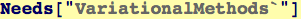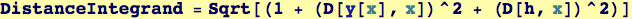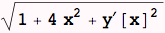Calling VariationalD from the package "VariationalMethods"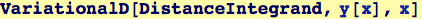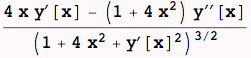Setting the variational derivative to zero is the Euler equation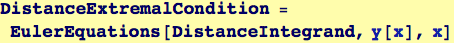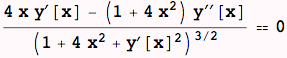The Euler equation is an ODE; this one has a closed-form solution.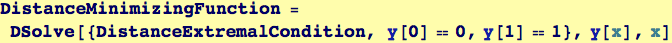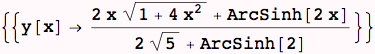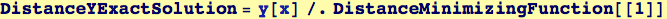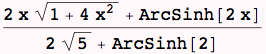Created by Wolfram Mathematica 6.0  (26 November 2007)